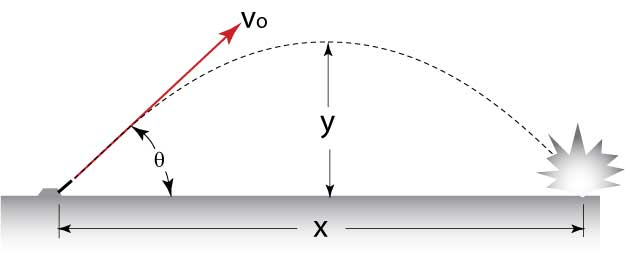# Projectiles aren't as easy as you might thinkA ball is thrown from ground With a velocity $v_{0}$, and at an angle $\theta$. With what angle $\theta$ should the ball be thrown to give it maximum arc length or maximum distance for its trajectory to cover? If $\theta$ is the maximum value find:

$\left\lfloor \theta \right\rfloor$ in degree?

Details and Assumptions

Maximum range doesn't necessarily mean maximum arc length.

×

Problem Loading...

Note Loading...

Set Loading...